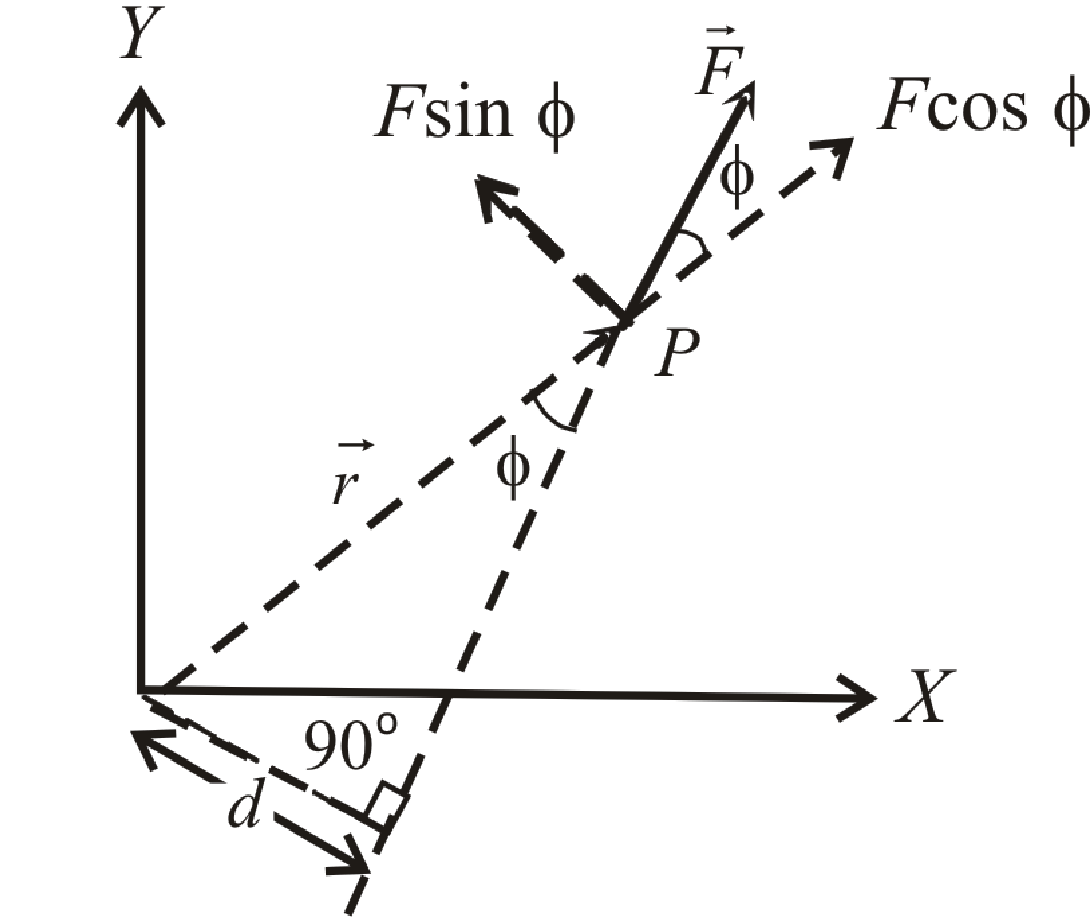BodhiAI
Oct. 31, 2019

#### Torque:

Torque:If the particle rotating in xy plane about the origin under the effect of forceand at any instant the position vector of the particle is, then

Torqueτ = rF sin φ

[whereφis the angle between the direction ofand]

(1) Maximum and minimum torque:

Asor τ = rF sin φ

 rmax. = rF When |sin φ| = max. = 1 i.e.  φ= 90°is perpendicular tormax. = 0 When |sin φ| = min. = 0 i.e.  φ= 0° or 180°is collinear to(2) If a body is acted upon by more than one force, the total torque is the vector sum of torques due to each force.(3) A body is said to be in rotational equilibrium if resultant torque acting on it is zero i.e.(4) Rotatory Motion :

TorqueWorkPowerTorque